# 大话原型链

1年前 ⋅ 515 阅读

Hello, 各位勇敢的小伙伴, 大家好, 我是你们的嘴强王者小五, 身体健康, 脑子没病.

## 前言## 何为原型链🐳 `__proto__`即为链表的 next 指针，链表尾部指针指向 null

``````class Person {};
let person = new Person();
``````🐳 `__proto__ -> constructor.prototype`

👺 原型链本质就是链表，__proto__即为next指针，指向其构造函数的原型🐢 坊间有一传闻，叫万物皆对象；就像下图，无论千变万化，链表的尾端始终为 Object.prototype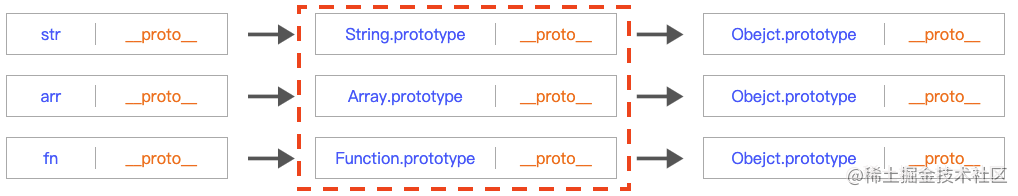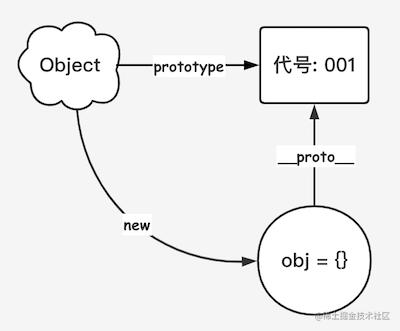obj`__proto__`指向其构造函数 Object 的原型 001

🚶 我们沿着原型链继续向前走，走到红框的位置，以 `str -> String.prototype -> Object.prototype -> null` 为例，图示如下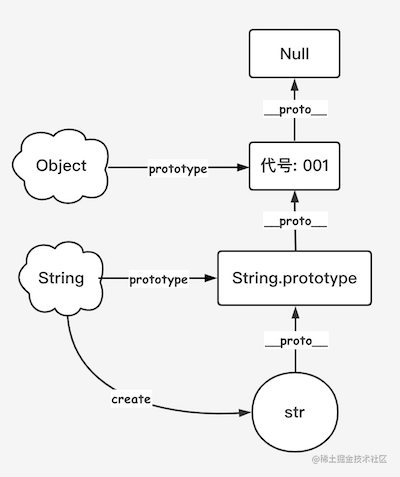``````let str1 = 'apple';
str1 instanceof Object // false

let str2 = new String('apple');
str2 instanceof Object // true
``````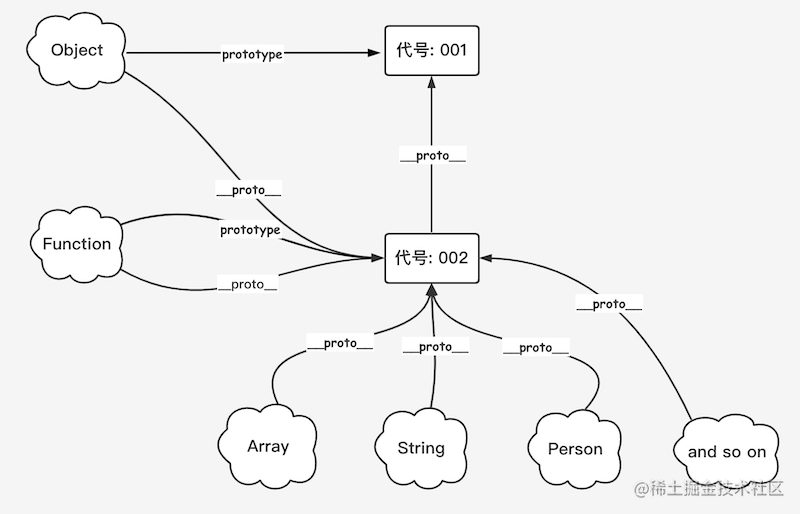## “new” 一个对象

👀 “new” 会发生什么呢? 不妨一起来看下

``````function Person (name) {
this.name = name;
this.action = function () {
console.log('打 🏓️ 乒乓球');
}
}

let person = new Person('黄刀小五');
``````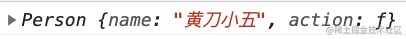new 的过程就类似于在链表头部插入元素， `__proto__` 指向其构造函数的原型。

``````function new (P, ...args) {
let obj = {};
obj.__proto__ = P.prototype;
P.call(obj, ...args); // 用于改变this指向, 使其指向P
return obj;
}

let person = new(Person, '黄刀小五'); // 输出同上
``````

## Object.create()

``````Object.Create = function (obj) {
let newObj = {};
newObj.__proto__ = obj;
return newObj;
}
``````

``````createObj -> obj -> Object.prototype -> null
``````

## 谈谈继承

``````function Teacher (name) {
this.name = name;
this.info = {};
this.action = function () {
console.log('做练习题');
}
};
``````

🐳 原型链继承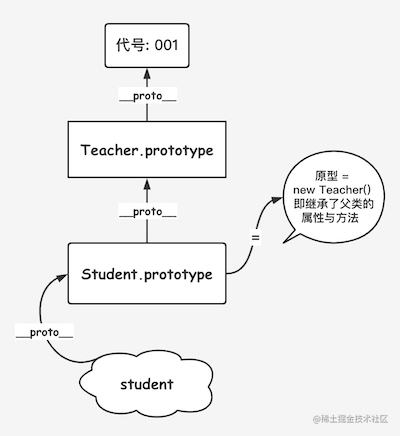``````function Student () {};
Student.prototype = new Teacher();
``````

Teacher 中有一 info 对象代表基本信息，我创建两个学生实例，如下，分别更改其 info 中的 age 会发生什么?

``````student1.info.age = 12;
student2.info.age = 13;

console.log(student1.info.age, student2.info.age); // 13 13
``````

🐳 构造函数继承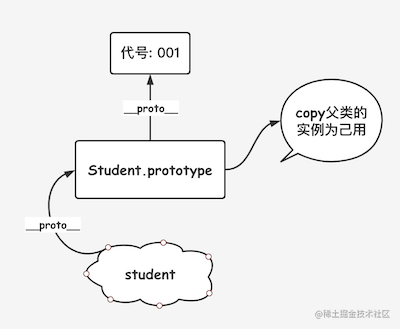``````function Student (...args) {
Teacher.call(this, ...args);
}
``````

``````Teacher.prototype.eat = function () {
console.log(`\${this.name} eat apple`);
}
``````

student 实例中调用下

``````const student = new Student('小五');
student.eat(); // student.eat is not a function
``````

🐳 组合继承

``````function Student (...args) {
Teacher.call(this, ...args);
}
Student.prototype = new Teacher();
Student.prototype.constructor = Student; // 重新赋值constructor, 否则其constructor为Teacher
``````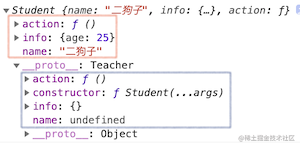🐳 原型继承

``````function create (o) {
let P = function () {};
P.prototype = o;
return new P();
}
``````

🐳 寄生式继承

``````function createStudent (o) {
let clone = create(o);
clone.study = function () {};
return clone;
}
``````

🐳 寄生组合式继承

``````function Student (...args) {
Teacher.call(this, ...args);
}
Student.prototype = Object.create(Teacher.prototype);
Student.prototype.constructor = Student;
``````

## 手写 instanceof

``````const instanceof = (L, P) => {
if (typeof L !== 'object') return false;

let current = L.__proto__;
while (current) {
if (current === P.prototype) return true;
current = current.__proto__;
}
return false;
}

instanceof('str', String) // false
instanceof({}, Object) // true
``````

## 小试牛刀

👺 No.1

``````Function.prototype.a = () => {
console.log(1);
}
Object.prototype.b = () => {
console.log(2);
}
function A() {};
const a = new A();

a.a();
a.b();
A.a();
A.b();
``````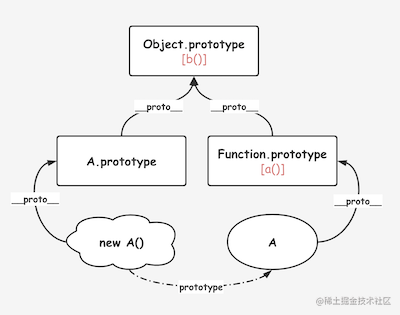``````a.a(); // Error
a.b(); // 2
A.a(); // 1
A.b(); // 2
``````

👺 No.2

``````var A = function() {};
A.prototype.n = 1;
var b = new A();
A.prototype = {
n: 2,
m: 3
}
var c = new A();

console.log(b.n);
console.log(b.m);

console.log(c.n);
console.log(c.m);
``````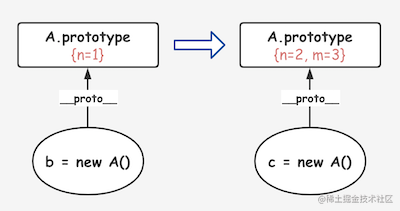``````console.log(b.n); // 1
console.log(b.m); // undefined
console.log(c.n); // 2
console.log(c.m); // 3
``````

👺 No.3

``````function A() {};
A.prototype.n = 0;
this.n += 1;
}

a = new A();
b = new A();
``````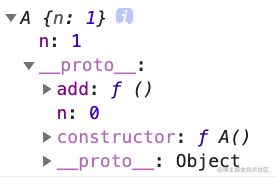``````console.log(b.add()); // 1
``````

## 参考🔗链接

[manxisuo] JavaScript 世界万物诞生记

[木易杨说] JavaScript常用八种继承方案

我有话说: